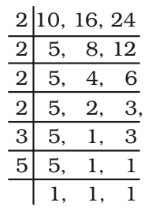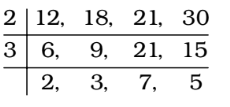## LCM and HCF

#### LCM and HCF

1. If the students of a class can be grouped exactly into 6 or 8 or 10, then the minimum number of students in the class must be

1. If the students of a class can be grouped exactly into 6 or 8 or 10, then
Required number of students = LCM of 6, 8, 10

##### Correct Option: B

If the students of a class can be grouped exactly into 6 or 8 or 10, then
Required number of students = LCM of 6, 8, 10 = 120

1. The smallest square number divisible by 10, 16 and 24 is

1. We find LCM of = 10, 16, 24∴ LCM = 22 × 22 × 3 × 5
∴ Required number = 2 × 2 × 2 × 2 × 3 × 3 × 5 × 5

##### Correct Option: D

We find LCM of = 10, 16, 24∴ LCM = 22 × 22 × 3 × 5
∴ Required number = 2 × 2 × 2 × 2 × 3 × 3 × 5 × 5
Required number = 3600

1. Which is the least number which when doubled will be exactly divisible by 12, 18, 21 and 30 ?

1. We have , The LCM of 12, 18, 21, 30∴ LCM = 2 × 3 × 2 × 3 × 7 × 5 = 1260

##### Correct Option: C

We have , The LCM of 12, 18, 21, 30∴ LCM = 2 × 3 × 2 × 3 × 7 × 5 = 1260

 ∴ The required number = 1260 = 630 2

1. Let the least number of six digits which when divided by 4, 6, 10, 15 leaves in each case same remainder 2 be N. The sum of digits in N is :

1. LCM of 4, 6, 10, 15 = 60
Least number of 6 digits = 100000
The least number of 6 digits which is exactly divisible by 60 = 100000 + (60 – 40) = 100020
∴ Required number (N) = 100020 + 2 = 100022

##### Correct Option: B

LCM of 4, 6, 10, 15 = 60
Least number of 6 digits = 100000
The least number of 6 digits which is exactly divisible by 60 = 100000 + (60 – 40) = 100020
∴ Required number (N) = 100020 + 2 = 100022
Hence, the sum of digits = 1 + 0 + 0 + 0 + 2 + 2 = 5

1. The least number, which when divided by 4, 5 and 6 leaves remainder 1, 2 and 3 respectively, is

1. We know that when a number is divided by a, b or c leaving remainders p, q or r respectively such that the difference between divisor and remainder in each case is same i.e., (a – p) = (b – q) = (c – r) = t (say) then that (least) number must be in the form of (k – t), where k is LCM of a, b and c .
Here t = 4 – 1 = 3, 5 – 2 = 3, 6 – 3 = 3

##### Correct Option: A

We know that when a number is divided by a, b or c leaving remainders p, q or r respectively such that the difference between divisor and remainder in each case is same i.e., (a – p) = (b – q) = (c – r) = t (say) then that (least) number must be in the form of (k – t), where k is LCM of a, b and c .
Here t = 4 – 1 = 3, 5 – 2 = 3, 6 – 3 = 3
∴ The required number = k - t = LCM of ( 4, 5, 6 ) – 3
Hence , The required number = 60 – 3 = 57